GLOSSARY OF TERMS USED IN PHYSICAL ORGANIC CHEMISTRY
(IUPAC Recommendations 1994)

# E

Continued from terms starting with D

Contents

ET-value

+ educt

Term used mainly in the German literature for starting material (reactant). It should be avoided in English, because there it means "something that comes out" and not "something that goes in". The German use of the term is in fact also incorrect.

effective charge

Change in effective charge is a quantity obtained by comparison of the polar effect of substituents on the free energies of rate or equilibrium processes with that on a standard ionization equilibrium. Provided the effective charge on the states in the standard equilibrium are defined, then it is possible to measure effective charges for states in the reaction or equilibrium under consideration. WILLIAMS (1984, 1992).

effective molarity (or effective concentration)

The ratio of the first-order rate constant of an intramolecular reaction involving two functional groups within the same molecular entity to the second-order rate constant of an analogous intermolecular elementary reaction. This ratio has the dimension of concentration. The term can also apply to an equilibrium constant. See also intramolecular catalysis.

eighteen-electron rule

An electron-counting rule to which an overwhelming majority of stable diamagnetic transition metal complexes adhere. The number of nonbonded electrons at the metal plus the number of electrons in the metal-ligand bonds should be 18. The 18 electron rule in transition metal chemistry is a full analogue of the "Lewis octet rule".

electrocyclic reaction

A molecular rearrangement that involves the formation of a sigma bond between the termini of a fully conjugated linear pi-electron system (or a linear fragment of a pi-electron system) and a decrease by one in the number of pi bonds, or the reverse of that process. For example: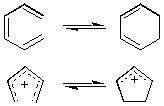The stereochemistry of such a process is termed "conrotatory" or antarafacial if the substituents at the interacting termini of the conjugated system both rotate in the same sense, e.g.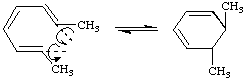or "disrotatory" (or suprafacial) if one terminus rotates in a clockwise and the other in a counter-clockwise sense, e.g.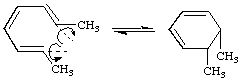electrofuge

A leaving group that does not carry away the bonding electron pair. For example, in the nitration of benzene by NO2+, H+ is the electrofuge. The adjective of electrofuge is electrofugal. See also electrophile, nucleofuge.

electromeric effect

A molecular polarizability effect occurring by an intramolecular electron displacement (sometimes called the "conjugative mechanism" and, previously, the "tautomeric mechanism") characterized by the substitution of one electron pair for another within the same atomic octet of electrons. It can be indicated by curved arrows symbolizing the displacement of electron pairs, as in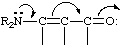which represents the hypothetical electron shift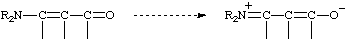The term has been deemed obsolescent or even obsolete (see mesomeric effect, resonance effect). It has long been custom to use phrases such as "enhanced substituent resonance effect" which imply the operation of the electromeric effect, without using the term, and various modern theoretical treatments parametrize the response of substituents to "electronic demand", which amounts to considering the electromeric effect together with the inductomeric effect. EHRENSON, BROWNLEE and TAFT (1973); TAFT and TOPSOM (1987); CHARTON (1987).

electron acceptor

(1) A substance to which an electron may be transferred; for example 1,4-dinitrobenzene or the dication 1,1'-dimethyl-4,4'-bipyridyldiium .

+ (2) A Lewis acid. This usage is discouraged.

electron affinity

The energy released when an additional electron (without excess energy) attaches itself to a molecular entity (usually an electrically neutral molecular entity). (The direct measurement of this quantity involves molecular entities in the gas phase.)

electronation

electron attachment

The transfer of an electron to a molecular entity, resulting in a molecular entity of (algebraically) increased negative charge. (It is not an attachment, as defined in this Glossary.)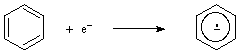electron configuration

electron-deficient bond

A single bond between adjacent atoms that is formed by less than two electrons, as in B2H6: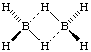The B-H-B bonds are called a "two-electron three-centre bonds".

electron density

If P(x,y,z) dx dy dz is the probability of finding an electron in the volume element dx dy dz at the point of a molecular entity with coordinates x,y,z, then P(x,y,z) is the electron density at this point. For many purposes (e.g., X-ray scattering, forces on atoms) the system behaves exactly as if the electrons were spread out into a continuously distributed charge. The term has frequently been wrongly applied to negative charge population. See also charge density.

electron detachment

The reverse of an electron attachment.

electron donor

(1) A molecular entity that can transfer an electron to another molecular entity, or to the corresponding chemical species.

+ (2) A Lewis base. This use is discouraged.

+ electron-donor-acceptor complex

electronegativity

A measure of the power of an atom or a group of atoms to attract electrons from other parts of the same molecular entity. The concept has been quantified by a number of authors, including especially Pauling and Mulliken. See ATKINS (1974).

electroneutrality principle

The principle expresses the fact that all pure substances carry a net charge of zero.

electronic effect of substituents: symbols and signs

The inductive effect has universally been represented by the symbol I. This is now commonly taken to include both through-bonds and through-space transmission, but I is also used specifically for through-bonds transmission; through-space transmission is then symbolized as F (for field effect). The symbols for the influence of substituents exerted through electron delocalization have variously been M (mesomeric), E (electromeric), T (tautomeric), C (conjugative), K (konjugativ), and R (resonance). Since the present fashion is to use the term resonance effect, R is the most commonly used symbol, although M is still seen quite often.

Both the possible sign conventions are in use. The Ingold sign convention associates electronegativity (relative to hydrogen atom) with a negative sign, electropositivity with a positive sign. Thus the nitro group is described as electron- withdrawing by virtue of its - I and - M effects; chloro is described as a - I, +M substituent, etc. For correlation analysis and linear free-energy relationships this convention has been found inconvenient, for it is in contradiction to the sign convention for polar substituent constants (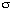-constants). Authors concerned with these fields often avoid this contradiction by adopting the opposite sign convention originally associated with Robinson, for electronic effects. This practice is almost always associated with the use of R for the electron delocalization effect: thus the nitro group is a +I, +R substituent; chloro a +I, - R substituent, etc.

electron-pair acceptor

A synonym for Lewis acid.

electron-pair donor

A synonym for Lewis base.

electron transfer

The transfer of an electron from one molecular entity to another, or between two localized sites in the same molecular entity. See also inner sphere (electron transfer), outer sphere (electron transfer), Marcus equation.

electron-transfer catalysis

The term indicates a sequence of reactions such as shown in equations (1)-(3), leading from A to B:

A + e-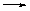A. - (1)

A. -B. - (2)

B. - + AB + A. - (3)

An analogous sequence involving radical cations (A.+, B.+) is also observed.

The most notable example of electron-transfer catalysis is the SRN1 (or T+DN+AN) reaction of aromatic halides.

The term has its origin in a suggested analogy to acid-base catalysis, with the electron instead of the proton. However, there is a difference between the two catalytic mechanisms, since the electron is not a true catalyst, but rather behaves as the initiator of a chain reaction. "Electron-transfer induced chain reaction" is a more appropriate term for the situation described by equations (1)-(3). EBERSON (1987).

electrophile, electrophilic

An electrophile (or electrophilic reagent) is a reagent that forms a bond to its reaction partner (the nucleophile) by accepting both bonding electrons from that reaction partner.

An "electrophilic substitution reaction" is a heterolytic reaction in which the reagent supplying the entering group acts as an electrophile. For example

C6H6 + NO2+ (electrophile)C6H5NO2 + H+ (electrofuge)

Electrophilic reagents are Lewis acids. "Electrophilic catalysis" is catalysis by Lewis acids.

The term "electrophilic" is also used to designate the apparent polar character of certain radicals, as inferred from their higher relative reactivities with reaction sites of higher electron density. See also electrophilicity.

electrophilicity

(1) The property of being electrophilic (see electrophile).

(2) The relative reactivity of an electrophilic reagent. (It is also sometimes referred to as "electrophilic power".) Qualitatively, the concept is related to Lewis acidity. However, whereas Lewis acidity is measured by relative equilibrium constants, electrophilicity is measured by relative rate constants for reactions of different electrophilic reagents towards a common substrate (usually involving attack at a carbon atom). See also nucleophilicity.

element effect

The ratio of the rate constants of two reactions that differ only in the identity of the element of the atom in the leaving group, e.g., kBr/kCl. As for isotope effects, a ratio of unity is regarded as a "null effect".

elementary reaction

A reaction for which no reaction intermediates have been detected or need to be postulated in order to describe the chemical reaction on a molecular scale. An elementary reaction is assumed to occur in a single step and to pass through a single transition state. IUPAC CHEMICAL KINETICS (1981). See also stepwise reaction.

elimination

The reverse of an addition reaction or transformation.

In an elimination two groups (called eliminands) are lost most often from two different centres (1/2/elimination or 1/3/elimination, etc.) with concomitant formation of an unsaturation in the molecule (double bond, triple bond) or formation of a new ring.

If the groups are lost from a single centre (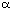-elimination, 1/1/elimination) the resulting product is a carbene or a "carbene analogue". See also-elimination.

enantiomer

One of a pair of molecular entities which are mirror images of each other and non-superimposable.

enantioselectivity

encounter complex

A complex of molecular entities produced at an encounter-controlled rate, and which occurs as an intermediate in a reaction mechanism. When the complex is formed from two molecular entities it is called an "encounter pair". A distinction between encounter pairs and (larger) encounter complexes may be relevant in some cases, e.g. for mechanisms involving pre-association.

encounter-controlled rate

A rate of reaction corresponding to the rate of encounter of the reacting molecular entities. This is also known as "diffusion-controlled rate" since rates of encounter are themselves controlled by diffusion rates (which in turn depend on the viscosity of the medium and the dimensions of the reactant molecular entities).

For a bimolecular reaction between solutes in water at 25 oC an encounter-controlled rate is calculated to have a second-order rate constant of about 1010 dm3 mol-1 s-1.

ene reaction

The addition of a compound with a double bond having an allylic hydrogen (the "ene") to a compound with a multiple bond (the "enophile") with transfer of the allylic hydrogen and a concomitant reorganization of the bonding, as illustrated below for propene (the "ene") and ethene (the "enophile"). The reverse is a "retro-ene" reaction.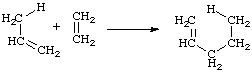energy of activation (Arrhenius energy of activation; activation energy), Ea (SI unit: kJ mol-1)

An operationally defined quantity expressing the dependence of a rate constant on temperature according to

Ea = RT2(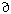ln k /T)p

as derived from the "Arrhenius equation", k = A exp(-Ea/RT), where A (SI unit: as for the corresponding rate constant) is termed the "pre-exponential factor". See also enthalpy of activation.

energy profile

enforced concerted mechanism

Variation of reaction parameters in a series of reactions proceeding in non-concerted steps may lead to a situation, where the putative intermediate will possess a lifetime shorter than a bond vibration, so that the steps become concerted. The transition state structure will lie on the coordinate of the More O'Ferrall-Jencks diagram leading to that of the putative intermediate.

enophile

See ene reaction.

entering group

An atom or group that forms a bond to what is considered to be the main part of the substrate during a reaction. For example: the attacking nucleophile in a bimolecular nucleophilic substitution reaction.

enthalpy of activation (standard entalpy of activation),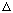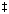Ho (SI unit: kJ mol-1)

The standard enthalpy difference between the transition state and the ground state of the reactants at the same temperature and pressure. It is related to the temperature coefficient of the rate constant according to the equation:H = RT2(ln k/T)p - RT = Ea - RT

= -R(ln(k/T)/(1/T))p

where Ea is the energy of activation, providing that the rate constants for reactions other than first-order reactions are expressed in temperature-independent concentration units (e.g., mol dm-3, measured at a fixed temperature and pressure). If lnk is expressed as

ln k = (a/T) + b + c ln T + dT,

thenH = -aR + (c - 1)RT + dRT2.

If enthalpy of activation and entropy of activation are assumed to be temperature independent, thenH = -aR

If the concentration units are mol dm-3, the true and apparent enthalpies of activation differ by (n - 1)/(RT2), where n is the order of reaction andthe thermal expansivity. See also entropy of activation, Gibbs energy of activation.

entropy of activation, (standard entropy of activation),So (SI unit: J mol-1 K-1)

The standard entropy difference between the transition state and the ground state of the reactants, at the same temperature and pressure.

It is related to the Gibbs energy of activation and enthalpy of activation by the equationsS = (H -G)/T

=H/T - R ln (kB/h) + R ln (k/T)

or, if ln k is expressed as ln k = a/T + b + c ln T + dT,S = R [b - ln (kB/h) + (c - 1)(1 + ln T) + 2 dT]

provided that rate constants for reactions other than first-order reactions are expressed in temperature-independent concentration units (e.g., mol dm-3, measured at a fixed temperature and pressure). The numerical value ofS depends on the standard state (and therefore on the concentration units selected). If entropy of activation and enthalpy of activation are assumed to be temperature-independent,S = R[b - ln(kB/h)]

Strictly speaking, the quantity defined is the entropy of activation at constant pressure from which the entropy of activation at constant volume can be deduced.

The information represented by the entropy of activation may alternatively be conveyed by the pre-exponential factor A (see energy of activation).

epimer

A diastereoisomer that has the opposite configuration at only one of two or more tetrahedral "stereogenic" centres present in the respective molecular entity. IUPAC STEREOCHEMICAL GLOSSARY (1993).

epimerization

Interconversion of epimers by reversal of the configuration at one of the "stereogenic" centres.

equilibrium, chemical

Reversible processes (processes which may be made to proceed in the forward or reverse direction by the (infinitesimal) change of one variable, ultimately reach a point where the rates in both directions are identical, so that the system gives the appearance of having a static composition at which the Gibbs energy, G, is a minimum. At equilibrium the sum of the chemical potentials of the reactants equals that of the products, so thatGr =Gr0 + RT ln K = 0Gr0 = -RT ln K

The equilibrium constant, K, is given by the mass-law effect.

equilibrium control

equilibrium isotope effect

See isotope effect.

ET-value

excess acidity

excimer

An excited dimer, "non-bonding" in the ground state. For example, a complex formed by the interaction of an excited molecular entity with a ground state counterpart of this entity. IUPAC PHOTOCHEMICAL GLOSSARY (1992). See also exciplex.

exciplex

An electronically excited complex of definite stoichiometry, "non-bonding" in the ground state. For example, a complex formed by the interaction of an excited molecular entity with a ground state counterpart of a different structure. IUPAC PHOTOCHEMICAL GLOSSARY (1992). See also excimer.

excited state

State of a system with energy higher than that of the ground state. This term is most commonly used to characterize a molecule in one of its electronically excited states, but can also refer to vibrational and/or rotational excitation in the electronic ground state. IUPAC ANALYTICAL CHEMISTRY (1982).

extended Hammett equation

This term applies in a general way to any multiparametric extension of the Hammett equation. It is sometimes used specifically for a form of dual substituent-parameter equation in which the actual value of the correlated property P under the influence of the substituent X is used, rather than the value relative to that for X = H. An intercept term h corresponding to the value of P for X = H is introduced, e.g.

P =I +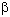R + h

The equation may be applied to systems for which the inclusion of further terms to represent other effects, e.g. steric, is appropriate. CHAPMAN and SHORTER (1972, 1978); CHARTON (1987).

external return

See ion-pair return.

extrusion transformation

A transformation in which an atom or group Y connected to two other atoms or groups X and Z is lost from a molecule, leading to a product in which X is bonded to Z, e.g.,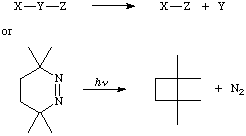The reverse of an extrusion is called insertion. See cheletropic reaction.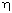-symbol ("eta")

See hapto.

References

*IUPAC STEREOCHEMICAL TERMINOLOGY (1993). IUPAC: Organic Chemistry Division: Basic Terminology of Stereochemistry. IDCNS and public review. Now published as Basic Terminology of Stereochemistry (IUPAC Recommendations 1996) in Pure Appl. Chem., 68, 2193-2222 (1996).

Continue with terms starting with F and G.